# VoltFormula tutorial

This tutorial shows you how to use VoltFormula in Volt Iris to convert formulas from OpenFormula and NotesFormula into Volt MX Go Rosetta Javascript. This feature in Volt MX Go Iris allows you to enter into source OpenFormula and Notes Formula Language and have it translated automatically into JavaScript code.

## Launch Volt MX Go Iris

1. Open Volt MX Go Iris. When the Sign-in dialog opens, close it.

Note

1. Go to Preferences.

For Windows, select EditPreferences.

For Mac, depending on your macOS, select Volt IrisPreferences or Settings.

2. On the Volt MX Go Iris Preferences dialog, click Volt MX Go Foundry.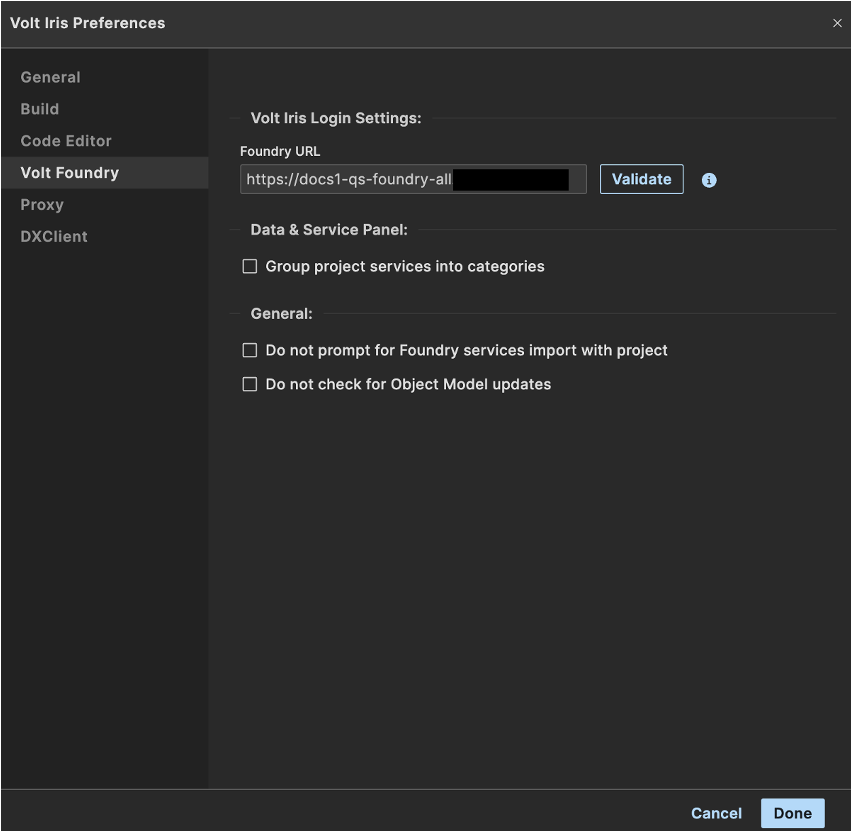3. On the Volt MX Go Foundry tab, enter your Foundry URL in the Foundry URL text box, and then click Validate.

You should see the “Validation Successful” message at the top of the dialog.

4. Click Done.

1. Click Login on the upper right corner of the Volt MX Go Iris screen.

## Open VoltFormula using the Action Editor

Tip

To see your Project, click in Design tab beside the Storyboard tab.

2. On the Project window, find the `Forms`. Once you click the `form`, the form appears on the canvass.
3. On the `form` canvass, select the widget or object you want to apply action to. Right-click it, and then select one of the action sequences, such as `onTouchStart`, `onClick` and others.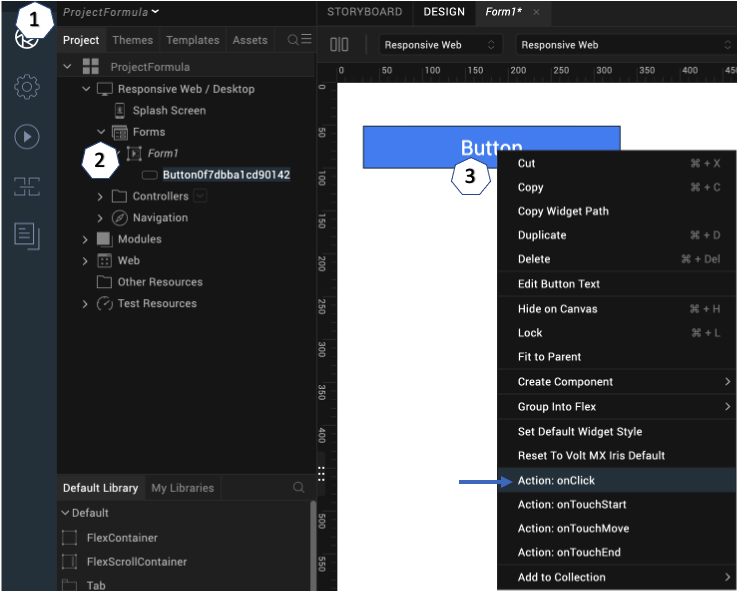The Action Editor opens and creates an action sequence for you to configure.

4. On the left side of the Action Editor, go to Formula and click the Add Formula.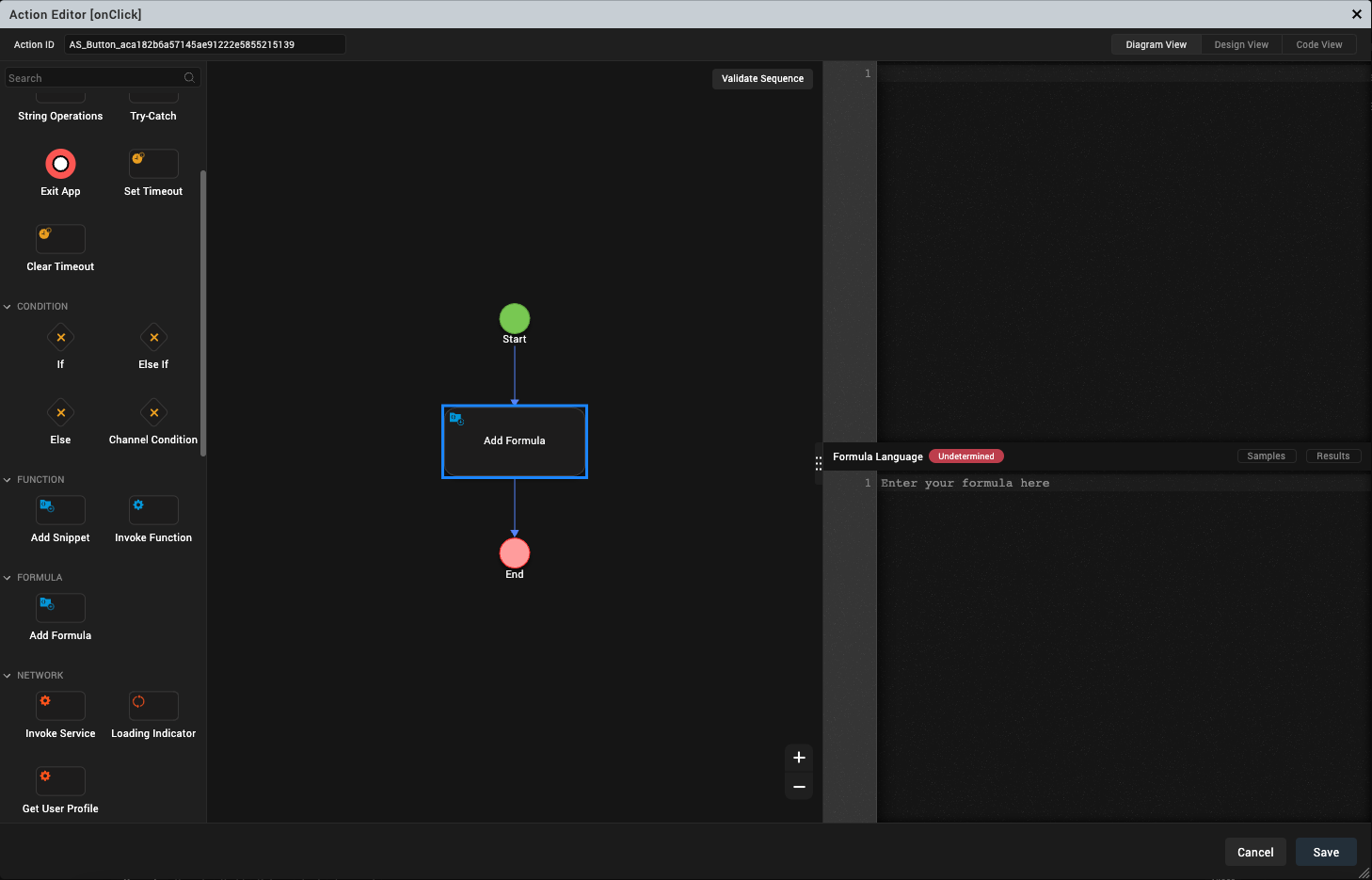## Translate OpenFormula and NotesFormula into Javascript

1. On the action editor, click the Add Formula on the diagram. On the right side of the action editor window, you can see the properties of the voltFormula.

• window for the translated OpenFormula and NotesFormula in JavaScript.
• window for the formula language - OpenFormula and NotesFormula are the accepted formulas to add in this window.
• window for the formula results.2. Click on Formula Language window and enter a valid formula.

Note

In Formula language window, it can detect the Notes and Open formula. You must enter the @ character before the formula in NotesFormula and enter the = character before the formula in OpenFormula.

Upon typing your formula, the intellisence will appear and return the syntax, descriptions, and examples of your chosen formula.

You can see automatically the JavaScript code with the formula you type in the Formula Language window.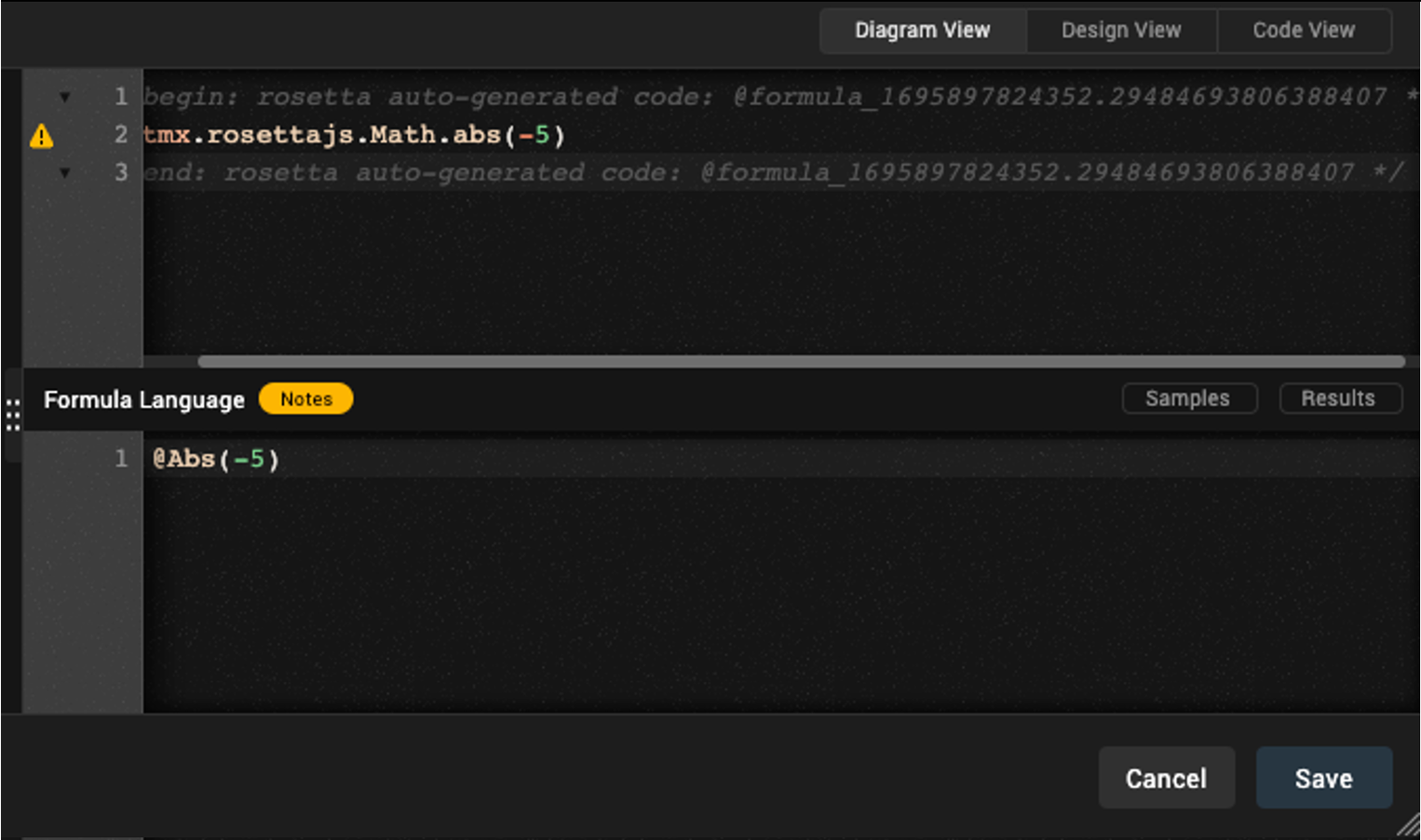You can also see the result upon clicking the Results button.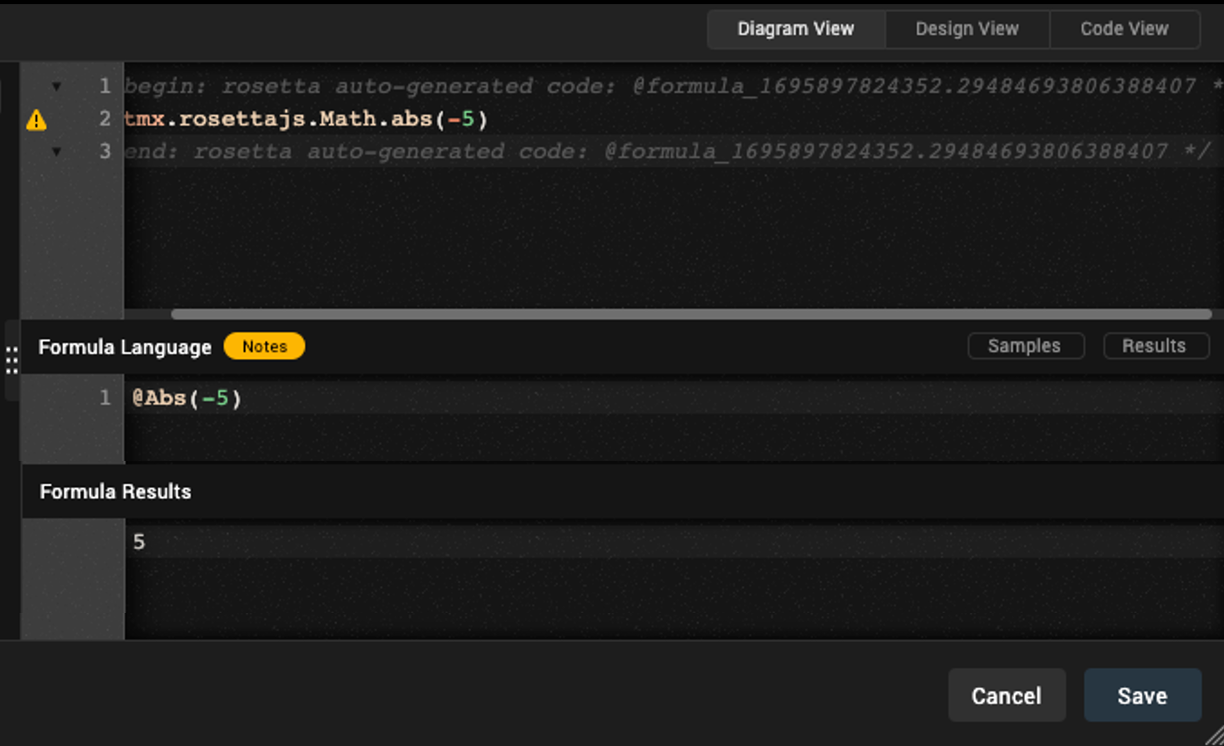3. Click Save.

## Use VoltFormula in a Controller module

### Add formula in a controller

1. Open a controller on Project Explorer.
2. Select and open your controller. This opens the code window.
3. Right-click the code window and select Add Code from Formula. The Formula Language window appear.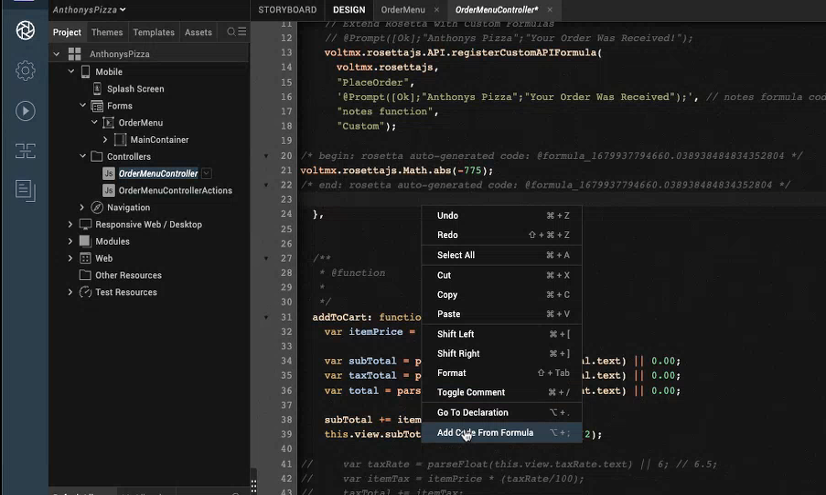4. Add the code in the Formula Language window.

Note

• @ character for NotesFormula
• = character for OpenFormula
• You can see the code hinting or the IntelliSense for the formula that you choose.
• Each formula is accompanied by its category.
• If you don't know how to convert the Javascript in Volt MX Go `rosettajs` from OpenFormula and NotesFormula, you may use the Formula Language window to do so.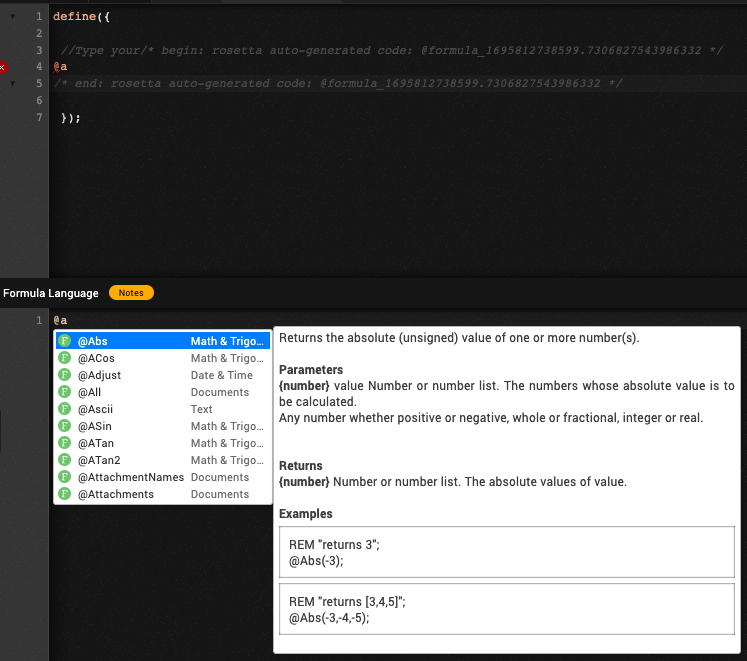The code you enter in Formula Language is translated to autogenerated rosettajs code in the code window.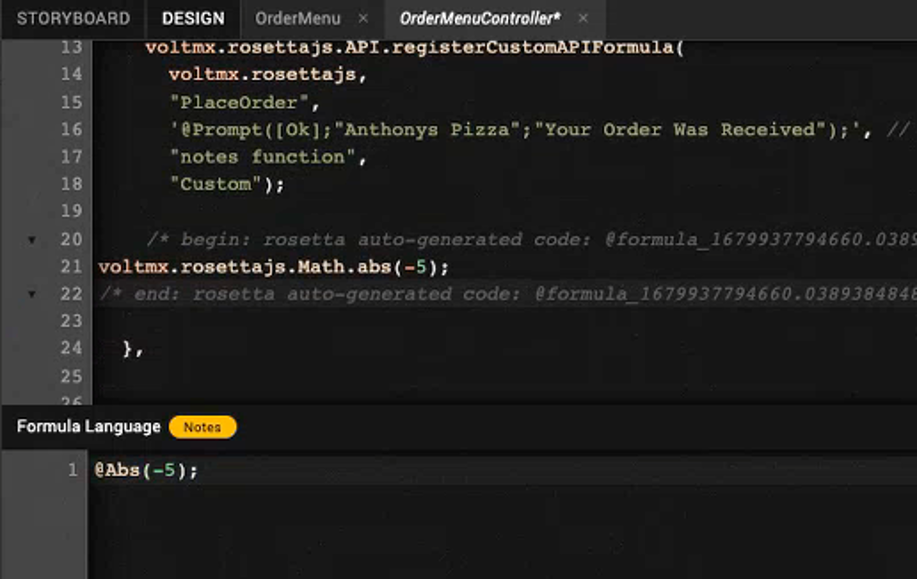### Edit formula in a controller

1. Open a controller on Project Explorer.
2. Select and open your controller. This opens the code window.

Note

You can see the conversion formula that comes from the OpenFormula and NotesFormula inbetween the comment of rosetta autogenerated code.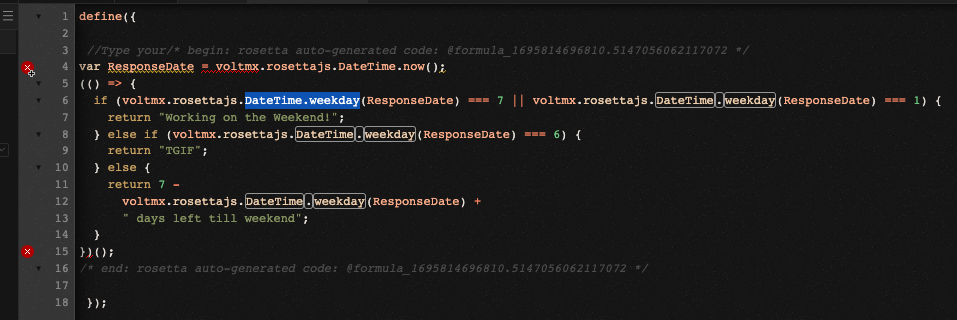3. Right-click the existing code and select Edit Formula Code. The Formula Language window appear and you can see the existing formula in the Formula Language window.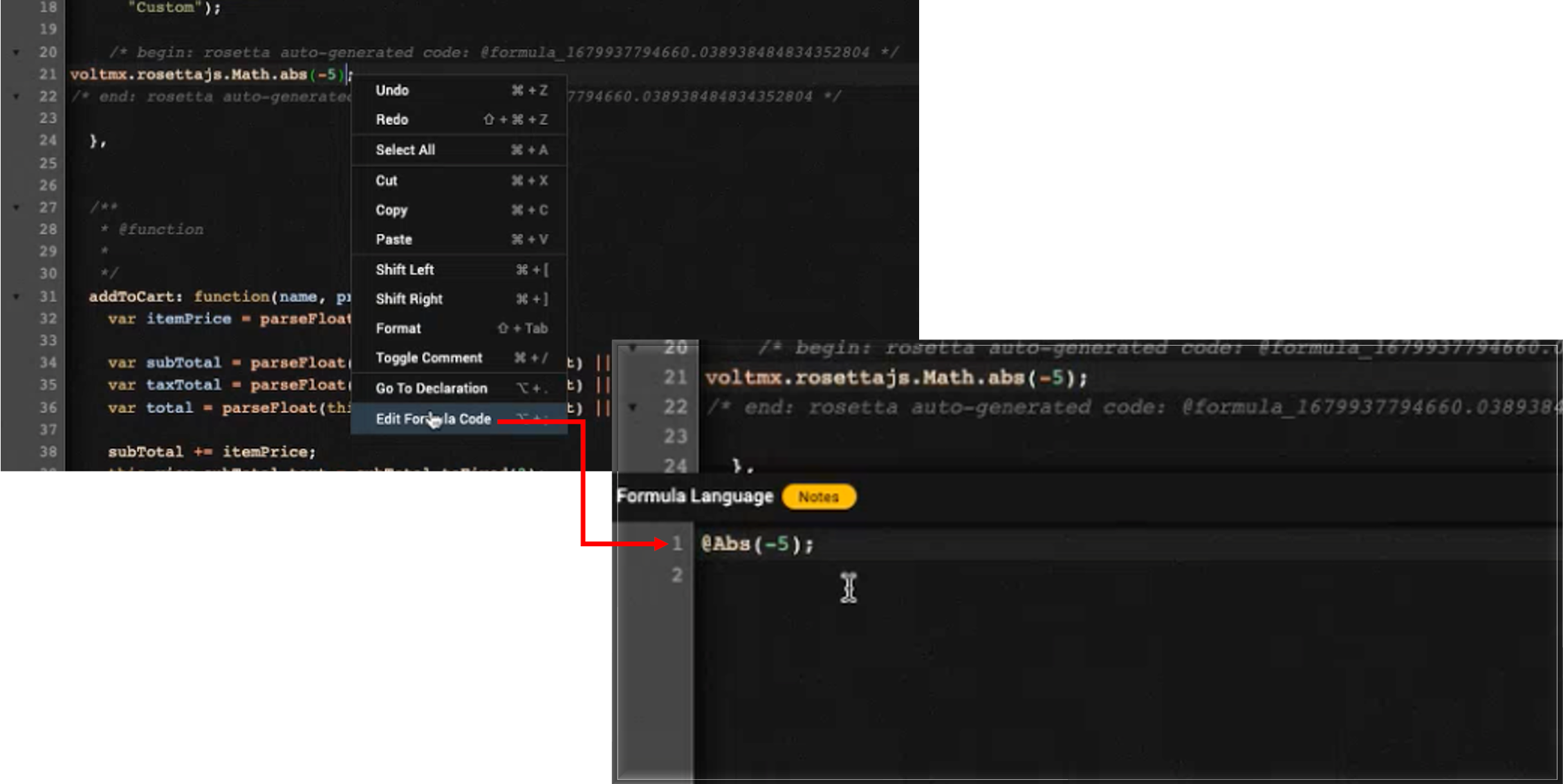4. You can edit the code you want in the Formula Language window.

Note

• @ character for NotesFormula
• = character for OpenFormula
• You can see the code hinting or the IntelliSense for the formula that you choose.
• Each formula is accompanied by its category.
• If you don't know how to convert the Javascript in Volt MX Go `rosettajs` from OpenFormula and NotesFormula, you may use the Formula Language window to do so.
• You can add or edit code in the formula language window as long as you follow the syntax.
• You can add more than one line of code.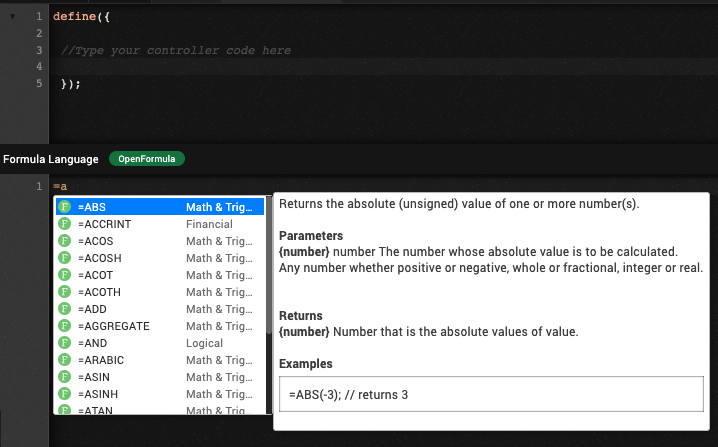Note

• The code you enter in Formula Language is translated to autogenerated `rosettajs` code in the code window.
• You can also see the result in Formula Results tab.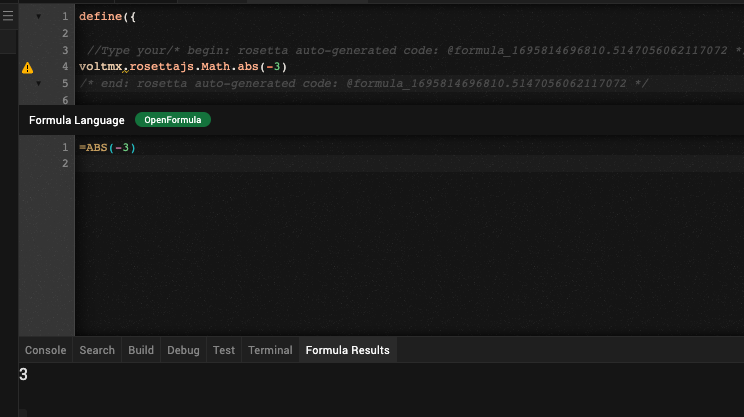### Edit Rosetta Javascript

1. Open a controller on Project Explorer.
2. Select and open your controller. This opens the code window.

Note

You can see the conversion formula that comes from the OpenFormula and NotesFormula inbetween the comment of rosetta autogenerated code.3. Add your formula in `rosettajs` in the code window, or edit the existing formula in the code window without opening the Formula Language window.

Note

• When you add `rosetta` javascript, you'll be able see the code hinting or the IntelliSense for the formula that you choose in code window.
• Make sure you know the syntax for coding the `rosetta` javascript.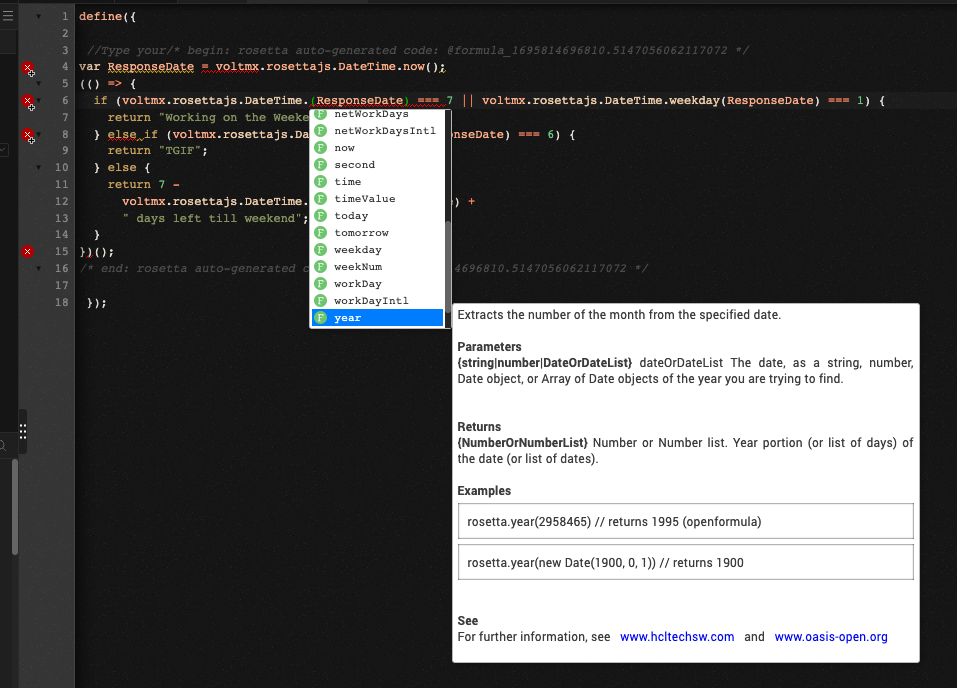Info

Last update: November 20, 2023